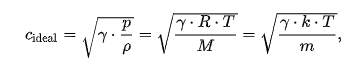# Speed of sound in an ideal gas

Gold Member
Homework Statement:
2.0kg of an ideal monoatomic gas(γ = 5/3) are contained inside a box of volume V=4.2m³.
Gas is at a pressure of 3.5bar.

-Calculate sound's velocity through the gas
Relevant Equations:
Speed of sound in a gas: ν=√(γkT/m)
IGE: pV=nRT
First of all I thought it was necessary to calculate the temperature(the only data missing for the formula) using the ideal gas equation(since I've already been given 'p' and 'V'), and plug it in the 'v' formula, but the problem immediately occurred when i tried to find out the number of moles(n), that I couldn't manage to find... and that's exactly where I got stuck.

Mentor
"monoatomic gas" means that your gas is composed of only one atom, or ##n=1##.
That is incorrect. Here n represents the number of moles of the gas present.

There is another formula for the speed of sound in a gas that involves pressure and density. Take a look at the wikipedia article on Speed of Sound.

•greg_rack
archaic
That is incorrect. Here n represents the number of moles of the gas present.

There is another formula for the speed of sound in a gas that involves pressure and density. Take a look at the wikipedia article on Speed of Sound.
Yes, I have deleted that comment, but somehow you were fasterLast edited:
•greg_rack
Gold Member
That is incorrect. Here n represents the number of moles of the gas present.

There is another formula for the speed of sound in a gas that involves pressure and density. Take a look at the wikipedia article on Speed of Sound.

Yeah, you're definitely right... I managed to solve the problem using the wikipedia formula "v = √(γ *p/d)", but I cannot understand why this works since in my physics book the only formula explained is "v = √(γ*k*T/m)", from which i cannot derive the resolving one!

MentorMore detail can be found on the Hyperhysics web page for the speed of sound in gases.

•greg_rack
Mentor
You're welcome!

•greg_rack